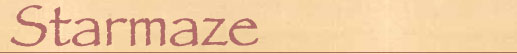Everyone knows that a square has 4 corners, but how many square faces are there in a tesseract? And how many tesseracts are there in a 9-cube? As an explorer of higher spaces, I often need to know the answers to arcane questions like this. Many years ago I found a quick and easy way of finding them: a triangle of numbers I call "Cartan's Triangle". And as we shall see, this triangle can open the door to many strange adventures.

If you have a copy of Excel, you can build your own Cartan's Triangle in less than 30 seconds, as follows:

1. Enter 1 in cell B1
2. Enter =A1+2*B1 in cell B2
3. Drag a selection rectangle as big as you want, from cell B2 to, say, cell K10
4. From the Edit menu, choose Fill Down then Fill Right
The result should look something like this: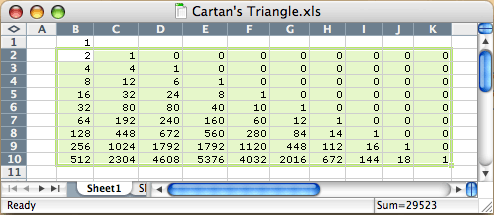Each row in this table represents an n-dimensional square. Row 1 is a point, row 2 is an edge, row 3 is a square, etc. The columns indicate the number of corners, edges, squares, etc. contained therein.

So, starting at row 1, we see that a point contains 1 "corner" and nothing else. Row 2 tells us that an edge contains 2 corners and 1 edge. Row 3 tells us that a square has 4 corners, 4 edges, and 1 square. And so on.

The answers to the above questions are now easy to find. Cell D5 tells us that a tesseract contains 24 squares. And cell F10 reveals number of tesseracts in a 9-cube: 4032.

Cartan's triangle works every time, and you can make one as big as you want. It seems magical that the interlocking complexities of endless higher-dimensional objects could all be revealed by a single, absurdly simple formula, B2 = A1 + 2*B1. Where did this formula come from?## Deriving the Formula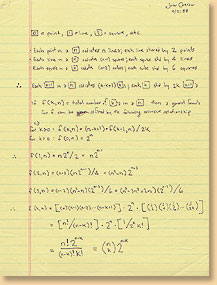By 1988 I realized that the starmaze was built along the edges of a nine-dimensional hypercube and I needed to know how these things were constructed. There are books that will tell you the number of squares in a tesseract, but I found that in order to really understand hypercubes I needed to reason these things out for myself.

One of the attractive things about higher dimensions is that it is quite possible to do this, even for those without a lot of mathematical training. I took a rather circuitous route, but had a lot of fun along the way. Here is how I did it.

First I invented a notation to represent the idea of an n-dimensional square:= a corner,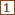= an edge,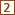= a square, etc.

I then coined the term "radiate" to describe the way multiple edges spring from a single corner or multiple squares start from the same edge. For me this captured the essence of how n-dimensional squares are constructed:

• Each corner in aradiates n edges; each edge shared by 2 corners
• Each edge in aradiates (n-1) squares; each square shared by 4 edges
• Each square in aradiates (n-2) cubes; each cube shared by 6 squares
When expressed in this way, it's easy to extend this sequence into the mysterious realm of higher dimensions. We can generalize this pattern into the following assertion:
• Eachin aradiates (n-k+1)'s; eachshared by 2k's
To check this out, try using 2 for k and 3 for n. The assertion then becomes:
• Each edge in a cube radiates 2 squares; each square shared by 4 edges
This is all very well and good, but it still doesn't tell me how many squares there are in a cube. What it does do, though, is tell me how squares radiate from edges. So if I knew how many edges there are in a cube, I could use my assertion to figure how many squares a cube has.

We know that each edge in a cube radiates (n-k+1) = 2 squares. So if a cube has 12 edges, there are a total of 12*2 = 24 squares radiated. This is still not right because we are counting each square more than once. To get the right number we have to divide this value by the total number of edges shared by each square. Fortunately, our assertion gives us that number as well: 2k, in this case 4. 24 / 4 = 6 squares, the correct answer.

So if we know the number of edges in a cube, we can figure out the number of squares it has. We can represent this mathematically by defining a recursive function. Let f (k,n) = the total number of's in awhere n >= k. Then:

f (k,n) = (n-k+1) * f (k-1,n) / 2k

For recursion to work correctly, we need to provide a stopping point. To find the number of squares in a cube, we need the number of edges. To find the number of edges, we need the number of corners. Fortunately, the number of corners in a cube (or any other n-square) is easy to figure out. There are 2 corners in an edge, 4 in a square, 8 in a cube, etc. The number of corners in an n-square is 2n.

So now we can fully define our function as follows:

For k>0, f (k,n) = (n-k+1) * f (k-1,n) / 2k
For k=0, f (k,n) = 2n

Now we're getting somewhere! Questions like "How many squares are there in a tesseract are now easy to answer:

f (0,4) = 24 = 16
f (1,4) = (4-1+1) * f (0,4) / 2*1 = 4 * 16 / 2 = 32
f (2,4) = (4-2+1) * f (1,4) / 2*2 = 3 * 32 / 4 = 24

A tesseract has 16 corners, 32 edges, and 24 squares. Still, recursive functions are a bit of nuisance. It would be better to have a straightforward formula that would give us the answer in one fell swoop. To figure that out, I tried leaving n as a variable and then plugging in successive values of k to see if I could generalize the pattern:

f (1,n) = n2n / 2 = n2n-1
f (2,n) = (n-1) (n2n-1) / 4 = (n2-n) 2n-3
f (3,n) = (n-2) (n2-n) (n2n-3) / 6 = (n3-3n2+2n)(2n-3) / 6

Aha! We can see a sequence forming here:

f (k,n) = [(n) (n-1) (n-2) ... (n-k+1)] * 2n * [(1 / 2) (1 / 4) (1 / 6) ... (1 / 2k)]

Using factorials we can simplify this to:

f (k,n) = [n! / (n-k)!] * 2n * [1 / 2kk!]

Or even better:

f (k,n) = n!2n-k / (n-k)!k!

Wait a second. n! / (n-k)!k! is the formula for (n choose k). So now all this gobble-de gook boils down to something surprisingly simple:

f (k,n) = (n choose k) * 2n-k

(n choose k) refers to the number of different ways of choosing k items from a set of n items. If you have four friends, Andy, Betty, Carl and Desdemona, but you can only invite 2 of them to join you for dinner, there are (4 choose 2) = 4! / 2!2! = 24 / 4 = 6 different ways of doing this:

1. Andy and Betty
2. Andy and Carl
3. Andy and Desdemona
4. Betty and Carl
5. Betty and Desdemona
6. Carl and Desdemona
In hindsight, it's not surprising that (n choose k) should show up in this formula. When you ask questions like "How many squares are there in a tesseract?" you are soon faced with the question "How many different ways are there to make a square using four dimensions?" A square is just an extension along any 2 of those 4 dimensions, or (4 choose 2).

So there are 6 basic ways of making a square in four dimensions, but each of these ways occur more than once. This is easier to see in 3 dimensions. Imagine yourself standing inside a cube-shaped room. There are (3 choose 2) or 3 different types of square:

1. YZ Squares perpendicular to the x dimension (which form the walls to your left and right)
2. XZ Squares perpendicular to the y dimension (which form the walls in front and behind you)
3. XY Squares perpendicular to the z dimension (which form the floor and ceiling)
Squares occur 2 times for each unused dimension. In a cube each square uses up 2 of the 3 available dimensions, so each type of square occurs twice. In a tesseract, each square uses up 2 of 4 available dimensions, so each of the 6 different types of square occurs 2*2 = 4 times. This is precisely what our formula tells us:

f (2,4) = (4 choose 2) * 24-2 = 6 * 22 = 24.## Triangles Old and NewWhen I discovered that (n choose k) was at the center of my formula, I remembered another place where this ubiquitous little function plays a prominent role: Pascal's Triangle. Pascal's triangle is a triangular array of numbers with many wonderful properties that make it a favorite tool of recreational mathematicians. It was published by Blaise Pascal in 1654, but had been discovered centuries earlier by both Chinese and Persian mathematicians.

It's easy to construct a Pascal's Triangle. Start with a "1" at the apex of triangle. Then put a "1" below and to its left and another "1" below and to its right to form the second row. The third row is "1 2 1". Each number is the sum of the number above and to the left and the number above and to the right (assume the space around the edge of the triangle is filled with zeros). When you are done, your triangle should look something like this:The numbers in Pascal's Triangle are binary coefficients. That is, the nth row of Pascal's Triangle starting with row 0) gives you the coefficients of (a + b)n. For example, row 3 of the triangle is "1 3 3 1" and (a + b)3 = a3 + 3a2b + 3ab2 + b3.

Binary coefficients show up all the time when you're dealing with hypercubes. Suppose you divide each dimension so that 30% of it is red and 70% of it is blue. A square built this way would consist of one small red square, two purple rectangles, and one large blue square - coefficients "1 2 1". A cube would consist of a small red cube, three beams, three slabs, and a large blue cube - coefficients "1 3 3 1". And so on into higher dimensions.So where does (n choose k) come into this? It turns out that the formula for the kth number (starting from 0) in the nth row of Pascal's Triangle is, you guessed it, (n choose k). The triangle works because

(n+1 choose k) = (n choose k-1) + (n choose k).

In thinking about this, I wondered if I could use the same trick to derive the numbers of my formula, f (k,n). I did the math and quickly discovered a very similar property:

f (k, n+1) = f (k-1, n) + 2*f (k, n)

This meant that I could make a triangle of my own. It would work just like Pascal's Triangle, but instead of deriving each value by adding the two closest numbers above it, you derive each value by adding the number above-left to 2 times the number above-right. The result looks like this:I am certainly not the first person ever to think of this. The formula for f (k, n) is widely known, and others have noticed that the numbers could be arranged in a triangle. But I had to call it something, and since no one else I know of bothered to name it, I decided to call my creation "Cartan's Triangle." I've found it very handy over the years and, as we've seen, you can use Excel to generate one from scratch in just a few seconds.

But wait. There's more!## The Third PowerThe rows of Pascal's Triangle sum to powers of two: 1, 2, 4, 8, etc. So after creating Cartan's Triangle, one of the first things I did was to sum each row. The values I got surprised me: 1, 3, 9, 27, 81, etc. These, of course, are powers of three.

By this time I had spent a decade wandering the starmaze, and had encountered powers of two at every turn. I could speak binary in my dreams. But in all that time I had never before encountered powers of three. Would I now have to learn to speak, uh, trinary?

As I studied the triangle it dawned on me that the answer was YES! If you really want to understand what a hypercube is made of, it would be handy to speak trinary.

Each row of Cartan's Triangle represents an n-dimensional square: a point, an edge, a square, a cube, a tesseract, etc. And each value in a given row represents a component of that n-square. Row 3, for example, tells me that a cube consists of 8 corners, 12 edges, 6 squares, and 1 cube. 8 + 12 + 6 + 1 = 33 = 27.

So if you wanted to index all the components of an n-square, and assign a unique number to each one, the numbers would always total to a power of three. I had been using binary numbers to index the corners of n-squares for years. I now saw that I could use the same approach to index all the components of an n-square. The most elegant way of assigning those numbers would be to give each one an n-digit trinary number.

Not only would this system assign a unique number to every component, it would also provide a meaningful description of that component. A trinary index number would be like DNA - even in isolation it would reveal exactly what kind of object it was, both type and subtype, and exactly where it lived in n-dimensional space. I saw how it would work in a flash and quickly filled a page with a definition:A Notational System For Uniquely Identifying
All Components Of An N-Dimensional Square
• Each component is assigned an n-digit base 3 number, with the right-most digit representing the x dimension, the next digit the y dimension, etc.

• The three symbols used are 0, 1, and X.

• A 0 indicates that the component is located at the origin of that digit's dimension.

• A 1 indicates that the component is displaced from the origin of that digit's dimension.

• An X indicates that the component is extended across that digit's dimension.

• Regardless of dimension, all n-squares have one corner located at the origin. That corner is always assigned the value (0,0,0,...,0) where the number of 0's is equal to the value of n.

• For example, the four corners and four edges of a square would be assigned the following values: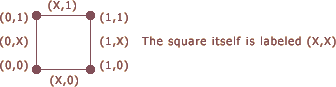• The number of X's indicates the type of component: no X's indicates a point, one X indicates an edge, two X's indicates a square, etc.

• The pattern of X's indicates the sub-type. For example, a 3-digit index containing two X's is a square component of a cube. There are three possible sub-types of such a square: a left/right yz-square, a back/front xz-square, and a bottom/top xy-square. Each sub-type has a corresponding pattern. A yz-square has X's in the 2nd and 3rd positions, a xz-square in the 1st and 3rd, and an xy-square in the 1st and 2nd.

• The points can be thought of as being located in an n-dimensional binary coordinate system with only two positions allowed along each dimension: located at the origin (0) or displaced from it (1). The coordinates can be represented by an n-bit binary number. Regardless of dimension, adjacent coordinates always differ by a single bit.

• Each subtype can be assigned a relative location by forming all non-X digits into an (n-k) bit binary number where k is the dimensional size (number of X's) of the component type. This can be thought of as collapsing all occurrences of a component to individual points by removing the k dimensions in which they extend and locating those points in an (n-k) dimensional binary coordinate system.

• To find the (k-1) dimensional sub-components which form the boundaries of a particular k-dimensional component, hold the non-X digits fixed and, for each X, change the X to a 1, then to a 0. This defines the two sub-components for each of the k X's in the component.## The Components of a CubeThe best way to understand this notational system is to try it out on a familiar object. The cube, with its 8 corners, 3 kinds of edge (4 each for a total of 12), and 3 kinds of square (2 each for a total of 6), is rich enough in components to fully illustrate the power of trinary indexing.

For each of the 27 components, I list its base 10 number, its 3-digit trinary number, and its type and subtype. There are three sub-types of edge: width edges extend along the x dimension, length edges extend along the y dimension, and height edges extend along the z dimension. Each of the three sub-types of square occur twice: there is a left and right yz-square, a back and front xz-square, and a bottom and top xy-square.

Base 10 zyx Component
0.000Corner (origin)
1.001Corner
2.00XWidth Edge
3.010Corner
4.011Corner
5.01XWidth Edge
6.0X0Length Edge
7.0X1Length Edge
8.0XXXY-Square (bottom)
9.100Corner
10.101Corner
11.10XWidth Edge
12.110Corner
13.111Corner
14.11XWidth Edge
15.1X0Length Edge
16.1X1Length Edge
17.1XXXY-Square (top)
18.X00Height Edge
19.X01Height Edge
20.X0XXZ-Square (back)
21.X10Height Edge
22.X11Height Edge
23.X1XXZ-Square (front)
24.XX0YZ-Square (left)
25.XX1YZ-Square (right)
26.XXXCube## The Nine-Space Hotel

Now that we have mastered the cube, we are finally ready to sail into deeper waters. Higher dimensional cubes quickly become a dizzying labyrinth of interlocking spaces. The trinary notation system is like finding a map to the maze.

Let's take a 9-cube for example. If we look at row 9 of Cartan's triangle we see that a 9-cube is composed of no less than 19683 components:

• 512 Corners
• 2304 Edges
• 4608 Squares
• 5376 Cubes
• 4032 Tesseracts
• 2016 5-Cubes
• 672 6-Cubes
• 144 7-Cubes
• 18 8-Cubes
• The 9-cube itself
I normally conceive of the starmaze as a set of 512 rooms (the corners of the 9-cube) connected by a total of 2304 one-way passages (the edges of the 9-cube). But let's try exploring the 9-cube in a different way. Let's try to imagine what it would be like to crawl through the cubes of the 9-cube. We are, after all, three-dimensional beings, and the rooms that we actually live in are cubes (more or less).

So let's start inside a particular cube, somewhere in 9-dimensional space. We can imagine it as a pleasant little room with a rug, a cozy couch, and maybe a few paintings on the walls. On a side table there sits a Tower of Hanoi puzzle with 3 disks on each of the 3 spindles and a piece if paper with a Tic Tac Toe game scribbled on it.

Each wall in this room includes a door leading to an adjacent cube. For added fun, there is a trap-door under the rug leading to lower room. And if we pull on the little chain dangling from the ceiling a wooden staircase folds down allowing us to climb up to the attic.

Everything seems perfectly normal at first. As we move from room to room, we find small differences: a red couch instead of a blue one, an odd piece of statuary, someone else's hat tossed casually on a small side-table. But each room has the same monotonous cubical shape with the same six exits.

The sheer size of the mansion we find ourselves in soon becomes oppressive. There seems to be no beginning or end to these odd little rooms. (There are, after all, over five thousand of them!)

And then the deja vu sets in. We stride confidently into a second room, then straight into a third, on into a fourth, and then, without ever changing direction, straight into the first room we left from. This becomes even more disconcerting when we climb into the attic, climb into the attic's attic, the attic's attic's attic, and then, puffing and panting, climb one more set of stairs, push aside a rug, and find... the very room we started from.

This is when the panic sets in.

Hollering for help only makes matters worse since we can hear our own yell coming at us from all six directions. So after a while we calm down and take a closer look at the room we are in. What's this? A tiny plaque mounted on the wall with the number 16152. How odd. Upon further inspection we find three more plaques mounted neatly in the center of each wall, each with a different number. It turns out that even the floor has its own number, and the ceiling too.

We get down on our hands and knees and peer into a corner. Sure enough, there is a very tiny number painted there, right on the baseboard. And following the edge up where the two walls meet we find yet another number printed sideways. Every edge has its own number, though the ones along the ceiling are quite difficult to read.

Our invesigation is complete when we stand in the exact center of the room and examine the little chain hanging down from the ceiling. On the end of that chain is a plum-sized crystalline orb, which seems to shimmer inside with shifting squares. And inscribed on the surface of that orb, with exquisite delicacy, we find the words: "The Nine-Space Hotel, Room 16179".

Now it the time to whip out our handy Cartan's Triangle. Left yours in your other coat pocket? Not to worry - you can always make another one. All you need is a pencil, a paper, and the ability to add.

Using the insight gained by your Cartan's Triangle, you begin by converting each number from base ten to base three. 16179 becomes X1101X0X0. This tells us that this particular room extends along the second, fourth, and ninth dimensions, even though the room still seems to our 3-D eyes to occupy the usual width, length, and height. Now, at least, we know where we are.

We can also find all the components of our current cube. The three X's in the cubes address represent extensions in space: height (from bottom to top), length (from behind to in front), and width (from left to right). If we change the first X to a 0 we are essentially collapsing the height of the room and reducing it to the bottom face of the cube, that is, the floor. Changing it to a 1 instead produces the ceiling. The same can be done to the other two X's as well, as follows:

 Floor 01101X0X0 3057 Ceiling 11101X0X0 9618 Rear Wall X110100X0 16125 Front Wall X110110X0 16152 Left Wall X1101X000 16173 Right Wall X1101X010 16176

The 12 edge numbers are just as easy to find. To find the edge marking the intersection of two walls, compare each digit of their two addresses. If a digit is the same, retain it. If the digit is an X in one address and a 0 or 1 in the other, choose the non-X digit. The resulting address will have only one X. For example:

 Front Wall X1101 10 X0 16152 Left Wall X1101 X0 00 16173 Front Left Edge X1101 10 00 16146

That initial X tells us that this edge extends in only one dimension, our Z dimension, from floor to ceiling. If we change that X to a 0, we get the corner at the bottom of that edge; if we change it to a 1, we get the corner at the top. The resulting 8 corners give us the exact boundaries of our cube in 9 space:

 Bottom Rear Left Corner (0,1,1,0,1,0,0,0,0) 2997 Top Rear Left Corner (1,1,1,0,1,0,0,0,0) 9558 Bottom Front Left Corner (0,1,1,0,1,1,0,0,0) 3024 Top Front Left Corner (1,1,1,0,1,1,0,0,0) 9585 Bottom Rear Right Corner (0,1,1,0,1,0,0,1,0) 3000 Top Rear Right Corner (1,1,1,0,1,0,0,1,0) 9561 Bottom Front Right Corner (0,1,1,0,1,1,0,1,0) 3027 Top Front Right Corner (1,1,1,0,1,1,0,1,0) 9588

Thanks to Cartan's Triangle, we now know EXACTLY where we are. But there is even more information concealed in the address. The trinary address can also tell us what KIND of cube we are in.

We saw earlier that there were three different kind of squares in an ordinary cube, each occuring twice. In a 9-cube there are no less than 84 (9 choose 3) different kinds of cubes, each occurring 64 times. This is where the total number of cubes in a 9-cube comes from: 84 times 64 is 5376. We know that our particular cube is of the 9-4-2 type, since that's where the X's are. This may explain the changes in decor from room to room. Perhaps all 9-4-2 cubes have red couches.

We can also find our position in relation to the other 63 9-4-2 cubes. If we collapse dimensions 2, 4, and 9, the result will be a 6-dimensional space, with all the 9-4-2 cubes reduced to points occupying the 64 corners of a 6-cube. When we simulate this collapse by removing the three X's in our address, we are left with a 6-bit number: 110100 or 52. This means that our cube is on the 53rd corner of the 6-cube (since the numbering starts with 0), coordinates (1,1,0,1,0,0).

So now we know that our cube is the 53rd instance of a type 9-4-2 cube. And we also know the addresses of all the other 9-4-2 type cubes. We are finally ready to start traveling to other rooms.

It is at just this moment that we see a beautiful little girl wander into the room directly in front of us. She smiles mischievously at us but instead of walking through the door she reaches up to touch something next to the door. We have something on our side of the wall as well, a funny little black knob surrounded by seven colored dots.

We had noticed these before next to all the doors, but had assumed they were merely some odd kind of decoration. As the little girl touches this thing, it becomes clear that it is a switch of some kind, currently pointing to the green dot. And the knob on her side of the wall must be attached to ours, because as she twists her knob from green to blue, our knob moves as well.

As the knob switches from green to blue a distinct clicking sound is heard. At that precise moment something astonishing happens. The room we were just looking at changes in an instant. Suddenly it's a different room altogether as if we've flipped from one television channel to another.

And the little girl? She has vanished altogether. But she must be somewhere because the knob is still turning. Click - it points to teal and once again the room we see through the door changes, yet there is still no sign of the little girl.

The knob is still turning. Click. Click. Click. The knob jumps to the magenta dot, then to the yellow dot, then to the brown dot. Three more empty rooms come and go. And then there is a final click to the seventh dot, which is colored bright red. And this time something even stranger happens. This time our door disappears!

The knob is still there and has finally stopped turning, but the place which a moment earlier had held a wide archway with rich wooden trim was now a solid plaster wall with no trace of an outline or anything to suggest a doorway had ever been there. In the distance we can hear someone giggling and the sound of running feet.

Oh dear. This 9-cube is even spookier than we thought. In order to understand what just happened, it might be better to retreat for a moment to a simpler world, the relatively cozy world of the 4-cube, or "tesseract".## A Crooked House

The tesseract provides a gentle introduction to the art of moving from cube to cube within a higher dimensional space. One of the most well-known and entertaining descriptions of the insides of a tesseract comes from Robert Heinlein's 1940 short story "-And He Built A Crooked House", available in Clifton Fadiman's excellent anthology Fantasia Mathematica.

In this story an eccentric architect builds a home in the shape of an unfolded tesseract. When an earthquake causes the tesseract to fold back into its natural form, the architect's client and his wife, who are seeing the home for the first time, begin to have some very odd experiences.

In its unfolded state, this tesseract looks like an upside-down crucifix with sidebars extending in four directions. The garage and entrance is on the first floor, with stairs extending up to a central room which opens onto a kitchen, drawing room, lounge, and dining room. Above this is the master bedroom, and above that a study at the top of the tower. The floor plan of the house looks something like this: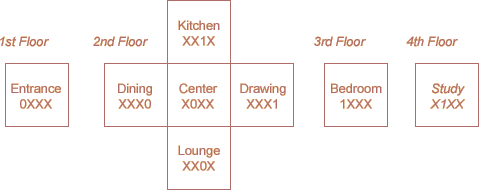I have assigned trinary index numbers to each room. If you study these numbers you will begin to get an idea of how the numbers change when you move from one room to another. The digits are in wzyx order, so the RIGHTmost position is the x direction (0=left, 1=right), the next digit is the y direction (0=back, 1=front), then z (0=bottom, 1=top). We'll save the weird w direction for later.

Every time you move from one room to another, one of the X's in the address changes places with a 0 or 1. You can think of this is a two-step process. First reduce one of the three X's to either a 0 or 1 - this is the equivalent of choosing which of the 6 walls you want to move towards. Then turn the other non-X digit into an X to step into the next room.

When you climb the stairs from the entrance to the center room, the X in the z position changes to a 0 and a new X appears in the w position. From the center, moving back or forth involves swapping the X in the y position, and moving left or right involves swapping the X in the x position. Another shift of the z position X takes us up to the third floor and another shift at the same position takes us to the top of the tower.

The easiest way to visualize a tesseract is as a small central cube with six pyramid stubs extending from each face and merging to form the six faces of a larger outer cube. That's how the central projection looks in 3-space; in 4-space the pyramid stubs are actually cubes and all eight cubes, even the "inner" and "outer" cubes, are the same size.

Here's where the weirdness begins. In the folded up tesseract, the master bedroom corresponds to that small inner cube. The center room is the pyramid extending from its bottom face and the kitchen, lounge, drawing room, and dining room are the north, south, east and west pyramids. The study is the final pyramid on the bedroom's upper face. And those six pyramids all converge to form the six faces of the inside-out entrance cube!

So if you go up from the 4th floor, you'll wind up on the 1st floor. In Heinlein's story, a window in the west wall of the lounge looks into the dining room from the south; this is because the west lounge wall and the south dining room wall are actually the same wall in 4-space. The floor of the kitchen forms the north wall of the entrance, its ceiling forms the north wall of the bedroom, and a window in the kitchen's north wall would look into the north wall of the study!

It's worth noting, by the way, that the tesseract is more than just these 8 cubes. The 8 cubes form the surface of the tesseract, just as 6 squares form the surface of a cube. But just as there is a whole space inside the surface of a cube, so there is a whole space inside the 8 cubes of a tesseract, a vaster and more mysterious space in which none of those 8 cubes partake. But let's get back to our cubes.

Every room address has 3 X's. By changing any one of these X's to a 0 or a 1, you can move in one of six directions, corresponding to the six faces of the cube. If we follow this simple logic, we can construct a complete chart or graph of all possible movements, with rooms as nodes and lines drawn whenever two rooms share a common wall. The resulting graph reveals the stunning symmetry of the tesseract:Heinlein's story includes a great chase scene as the architect pursues a mysterious stranger in a straight line through the drawing room, kitchen, dining room, and lounge without ever getting any closer to his prey. He then realizes that he has been chasing himself. This path appears in the above graph as a rectangle, as do other straight line circuits like the climb from entrance to center to bedroom to study and back to entrance.

Notice that in this diagram, each square represents a cube-shaped room, and each line represents a wall. Each room node has exactly six lines projecting from it, corresponding perfectly to the six square faces of a cube, for a total of 24 lines in all (8 rooms times 6 divided by 2, since otherwise each line would be counted twice). Each line connects two nodes, just as each wall connects two adjoining rooms.

This is exactly as one would expect and matches exactly with line 4 of Cartan's Triangle. As predicted, a tesseract has 24 squares (walls) and 8 cubes (rooms). But if you think that you now understand the way walls behave in higher spaces, you will be in for a shock. Patterns that seem clear and well-understood in one dimension are often utterly undone in the next. The journey from three dimensions to four was strange enough, but the journey to the fifth dimension is stranger still.## The 5-Cube

Let's have another look at Cartan's Triangle. This time, pay special attention to the diagonals indicating the number of walls and rooms in each higher space: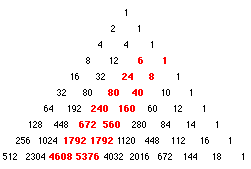Do you see what is happening? The number of rooms are increasing at a faster rate than the number of walls. By the time we get to the ninth dimension, there are more rooms than there are walls!

How can this be? The answer is that in dimensions higher than four, each wall is shared by more than two rooms. In the fifth dimension, each wall is shared by three rooms. A doorway in any wall connects rooms A, B, and C. So when you leave room A, you might walk into room B. But then again, you might walk into room C.

Actually, this same phenomenon did occur in Heinlein's story. The reason it happened in his tesseract and not ours is that we are treating our n-cubes as closed systems. In Heinlein's story, his tesseract also had connections to normal 3-space. In fact, he posited a space that curves just like a piece of paper might wrap around a cube. Flatlanders living on that piece of paper might enter the cube in one part of their "space", travel a short distance inside the cube, and exit on a piece of the paper that seems far away to them. In a similar fashion, one window of Heinlein's house looks out over Los Angeles while another looked straight down into Manhattan.

Heinlein handled this awkward situation by supposing that the choice about whether you move from A to B or A to C is controlled by your subconscious mind and appears essentially random. I find this unruly, so I suggest instead that we install little knobs next to each door so that we can consciously switch from one option to the other, like the channels of a TV set. I'd also prefer to do this in a symmetrical way, so that whenever room A is connected to room B, room B is also connected to room A.

My first attempt to do this was not entirely successful. I thought that since a given wall in room A could lead to either room B or room C, I would need a knob with two possible channel positions. The "black" channel would lead to B and the "red" channel would lead to C. The knobs controlling any given wall would always stay in synch.

I tried drawing a diagram of room connections just as we did for the tesseract. I knew going in that the diagram would be a tad more complicated: a 5-cube contains 40 rooms instead of 8, and since each wall now has a knob that lets you switch between two possible destinations, there are 12 lines projecting from each room instead of 6, for a total of 240 (40 * 12 / 2) lines. I found a symmetrical way of assigning two channels to each wall so that half the lines were black and half were red. The result looked like this (click to see the full diagram):What a mess! To get a better grasp of the underlying relationships, I next tried drawing only the black lines. This was much better. If you click this diagram you will see that the rooms are neatly partitioned into two sets of ten rooms (with 00 or 11 in their addresses) and one set of twenty rooms (with 01 or 10 in their addresses). In the larger partition, I turned the lines green whenever they connected a 10 to a 01:The diagram reveals that there are ten types of room inside the 5-cube, each occurring 4 times (00, 01, 10, and 11) for a total of 40 rooms. But there was still something that didn't add up. Recall that I said there were 240 connecting lines in the 5-cube diagram, half red and half black. If each wall leads from either A to B (black) or A to C (red), it seems like I should have 120 (240 / 2) walls. But if you look at Cartan's triangle, you will see that there are only 80 walls in a 5-cube. What did I do wrong?## The Right Colors

The number of channels per cube wall in an N-cube is N-3 (1 in a tesseract, 2 in a 5-cube, 3 in a 6-cube, etc.) And so the total number of cube-to-cube connecting lines in an N-cube diagram is simply the total number of cubes times the number of walls per cube (6) times the number of channels per wall (N-3) divided by 2 (so we don't count the same line twice).

According to Cartan's triangle, this number is increasing faster than the actual number of walls connecting the cubes. If we divide the number of connecting lines by the number of walls, we find a familiar sequence:

N Connections Walls C / W
4 24 24 1
5 240 80 3
6 1440 240 6
7 6720 672 10
8 26880 1792 15
9 96768 4608 21

This sequence (1, 3, 6, 10, 15, 21, ...) is simply a list of the triangular numbers (1, 1+2, 1+2+3, etc.). What did this mean? Something painfully obvious, no doubt.

I began using actual trinary addresses of particular walls and adjoining rooms, just as I did with room number 16179 above, to see exactly how all these things are connected in different higher dimensional spaces. I drew diagrams which showed exactly which rooms connected to which through a given wall.

These diagrams all turned out to be what is known as "complete" or "universal" graphs, that is, graphs in which each node is connected to every other node. And that's when the light bulb finally went on. The number of edges in a complete graph of order N is the Nth triangular number. When N=3 you have a triangle of 3 nodes and 3 edges. When N=4 you have 4 nodes and 6 edges. The reason the number of edges increases faster than the number of nodes is because the edges represent every possible combination of two nodes (N choose 2).

Here is the mistake I made. When I added channel-changing knobs to every wall of the 5-cube I assumed that each wall was doing double duty, connecting room 1 to either room 2 or room 3 (and 2 back to 1 and 3 back to 1) depending on the channel selected. But I forgot that this same wall ALSO connects 2 to 3.

So when you are standing in front of a doorway in room 1 of a 5-cube, not only does that door sometimes lead to room 2 and sometimes to room 3, sometimes there may be people that you can't even see using that same door to walk between room 2 and room 3! In order to make this work, my channel knobs would need more than just two colors.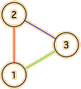In my diagrams, I used the color of an edge connecting two nodes (rooms) to represent the channel setting on the wall (from either side) when those two rooms are connected. In choosing colors for the edges, I had to make sure that no channel setting ever led to more than one room. The minimum number of colors needed to accomplish this is a value known in graph theory as the chromatic index.

• When n is even, the chromatic index of the complete graph on n nodes is n - 1
• But when n is odd, the chromatic index of the complete graph on n nodes is n
For any 3-cube in an N-cube, the number of channel positions for each wall (the number of edges per node) is N-3, and the number of nodes in the complete graph is N-2. The above rule about the chromatic index tells us that the number of colors to choose from will be N-3 if N is even or N-2 if N is odd.

For the tesseract (4-cube) things were deceptively easy: 1 channel and 1 color, which meant that channel selectors were not even needed. For the 5-cube, we need 2 channels per wall as expected, but the number of colors needed is 3.

The knob in room 1 will have two valid destination settings, say red and green. The knob in room 2 will also have two destination settings, but instead it will use red and blue. And the knob in room 3 will allow travellers to choose from either blue or green.

That's two channels per wall, but 3 colors (red, blue, and green). And since the three channel knobs in the three rooms are all controlling the same wall, each knob has to show all 3 colors. So if someone in room 2 changes the knob from red to blue, the knobs will change to blue in all three rooms.

This is fine for the people in rooms 2 and 3, who both have blue as one of their two valid destination settings. But room 1 only has red and green as valid destinations, so what happens when the knob in room 1 changes to blue? In that case, the door will disappear altogether, since the wall no longer connects room 1 to anywhere. This bizarre outcome only happens in odd-numbered N-cubes.

The same rules apply for higher dimensions. For the 6-cube we need 3 channels per wall but can use the same 3 colors we used in the 5-cube, so every color is a valid destination and there are no disappearing doors. In the 9-cube, which is where our story started, each door has a knob with 6 valid destination settings, but since 9 is an odd number, 7 colors are required and each knob will have a seventh color that makes its door go away.

The only remaining problem is finding the correct arrangement of colors for each value of N. Fortunately this is not too hard to do. The following chart sums up the colors needed for each N-cube. In each case, the diagram shows how the rooms sharing each wall are connected: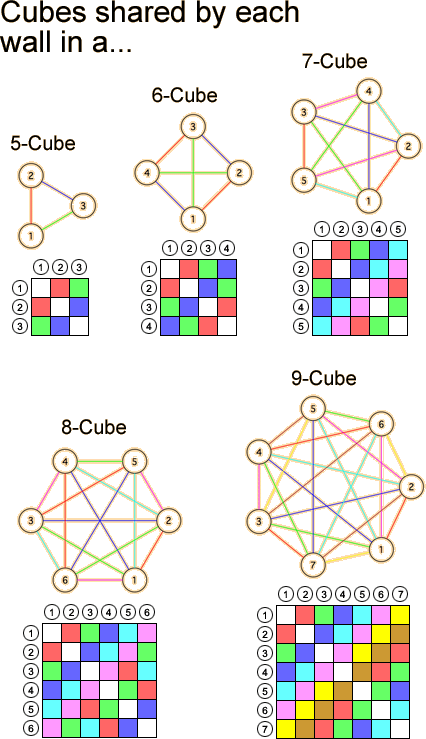For each chart I have chosen colors that are symmetrical (they reflect across the diagonal) and unique (no color appears more than once in any row or column). This ensures that if the red channel on a given wall connects room 1 to room 2, red will also connect room 2 to room 1 and there will be no other red channels on that wall. The colors can be assigned in a mechanical (but hard to describe) way that can be extended to any number of higher dimensions.

Now we finally have enough information to understand how that little girl in the Nine-Space Hotel made our door disappear. Room 16179 has the same channel knob as room 5 in the 9-cube diagram above. When we first encountered her, the little girl was standing in an adjacent room with a type=6 knob. We could see each other, which meant that wall must have been set to the green channel.

If you look at row 6 column 5 of the color chart (from the girl's point of view) or row 5 column 6 (from our point of view) you can see what happened each time she flipped the channel. When she flipped from green to blue, her door disappeared. But our door switched from room 6 to an empty room 7 - which is why the girl disappeared from our point of view.

She then flipped to the teal channel, which meant that she now was looking at room 7 and we were looking at room 1. Switching to magenta caused her to see room 1 and us to see room 2. She switched to yellow then brown. Now she was seeing 3 and we were seeing 4. Her final switch was to the red channel, which meant she was seeing room 4. But room 5 does not have a red destination, so now it was our turn for our door to disappear. The girl was free to run giggling into room 4 while we stared amazed at the place where our door has just been.

The color table also allows us to finally draw the correct diagram for the 5-cube, using three colors instead of two. Click the following close-up to see the full diagram in all its glory, with red, blue and green connecting lines instead of the black and red lines of my original attempt.The three rooms shown in this close-up, XX11X, XX1X1, and XXX11 all happen to share the same wall. The algorithm I used to assign edge colors is to first remove the two Xs in common to any two adjoining cubes, then, depending whether the remaining X is in the 1st, 2nd, or 3rd position, I assign it a 1, 2, or 3 and refer to the color chart to determine the edge color.

As you can see in this case, a red line connects XXX11 (room type 1) to XX1X1 (room type 2), a blue line connects XX1X1 to XX11X (room type 3), and a green line connects XX11X back to XXX11. This exactly matches the 3-room triangle diagram for the 5-cube above, and works perfectly for every other set of 3 rooms sharing a common wall as well.

The resulting diagram is just as messy as it was before. But, as before, we can gain a clearer picture of the 40 cubes depicted by restricting the edges to those of just one color. I did this for red, green, and blue colors and combined them into the following diagram (click to see it full-size).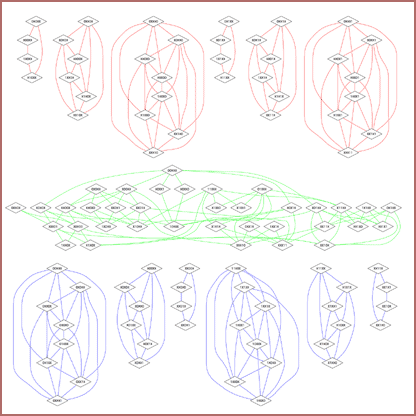As you can see, red and blue links both partition the rooms into six distinct sub-graphs, while green keeps everything fully connected. All three cases, though, only include 36 of the 40 cubes. There are 4 cubes with no red connections at all, 4 (different) cubes with no green connections, and 4 more with no blue connections.

Using this technique and the trinary index numbers derived from Cartan's triangle, we have created a complete map of the cubes in a 5-cube, which could be printed out and used to plot a path from any cube to any other. The same technique could be used to create just such a map of the Nine-Space Hotel, though with 5,376 rooms and 96,768 connecting lines of 7 colors, we would need a larger sheet of paper.## Tic Tac Toe

By showing you not just the finished results, but also my own mistakes and contortions along the way, I hope I have conveyed what it is like to explore higher spaces and how useful Cartan's triangle can be. Now, as a finale, I'd like to apply trinary indexing one more time to make a simple game more interesting.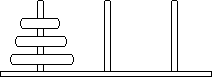There are a number of possible candidates for which trinary indexing is a natural fit. The first game that occurred to me was the Tower of Hanoi. The three spindles in this game can represent a 0, 1, and X. The disks placed on these spindles are digits which vary according to what spindle they reside on, with the digits ordered according to the size of the disk.

In this way, every possible position in the Tower of Hanoi puzzle can be assigned an N-digit trinary number where N is the number of disks. It is then possible to correlate every position of the puzzle with a particular component of an N-cube. Each move becomes the change of a single digit, which corresponds to moving a corner to a different location, or changing that corner to an edge, or changing that edge to a square.

If the starting spindle is "0" and the ending spindle is "1", the entire puzzle becomes one of finding a path from the origin (0,0,...,0) to the opposite corner (1,1,...,1). Whenever you are forced to use the third spindle, the component will increase in dimension, from a point, to an edge, to a square and so forth - and removing disks from the third spindle will have the reverse effect.

The solution to the puzzle, then, becomes an animation showing a point growing and hopping and shrinking and hopping and growing again, until it finally shrinks back down to a point. Looking at the puzzle in this way might help you see how to solve it, and adds an extra layer of meaning to every step along the way.

But on further consideration, I thought of an even simpler, even more familiar game that I could corrupt with my higher-dimensional hijinks: the venerable game of Tic Tac Toe.

Tic Tac Toe employs the same three-by-three grid used by the Starmaze. Each of its nine cells can be in one of three possible states: X, O, or empty. If we assign X cells the digit X, O cells the digit 0, and empty cells the digit 1, then each position in a Tic Tac Toe game can be reduced to a nine-digit trinary number.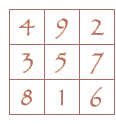Our next decision is what order to place the nine cells in. We can number the cells any way we want, but as is my custom, I prefer to use the Lo Shu. This ancient magic square adds an extra element of charm. So we'll place the rightmost digit (the x dimension) in the cell below the center, the next y dimension digit in the top right corner, etc.

Tic Tac Toe starts with an empty grid. This corresponds to a corner with coordinates (1,1,1,1,1,1,1,1,1) or 9841 in base 10, a point as far away from the origin as possible.

Every move that player O makes (let's call him Oscar) will change one of these 1s to a 0. This will simply move the referenced component one step closer to the origin. Every move that player X makes (let's call him Xavier) will change one of these 1s to an X. This will change the corner into an edge, the edge into a square, and so forth.

So Oscar and Xavier each seek to change the component in a different way. Oscar always tries to move the component closer to the origin. Xavier always tries to raise the component to a higher dimension.

The goal of the game is to reach a winning component that corresponds to three Os in a row for Oscar or three Xs in a row for Xavier. This component will be either a square (which can only happen if Oscar goes first and wins before Xavier can place his third X), a cube, a tesseract, or a 5-cube (which can only happen if Xavier goes first and wins by filling the last empty cell).

To see how this works, let's follow a typical game, step by step. We'll let Oscar go first.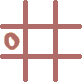First move. Oscar places an O in cell 3. The component has now shifted from 9841 to 9832 or 111111011. This is a corner one step closer to the origin in the z dimension.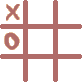Second move. Xavier responds by placing an X in cell 4. The component number is now 9859 or 11111X011. This is an edge along the w dimension extending from (1,1,1,1,1,0,0,1,1) to (1,1,1,1,1,1,0,1,1).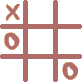Third move. Oscar places an O in cell 6, resulting in component 9616 or 11101X011. This effectively moves the edge one step closer to the origin along the 6th dimension.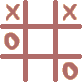Fourth move. Xavier extends the edge to a square by placing an X in cell 2. The component number is now 9619 or 11101X0X1. The square extends across the w and y dimensions.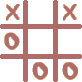Fifth move. Oscar, who is perhaps not the brightest bulb on the tree, responds by placing an O in cell 1. This subtracts 1 from the component number, changing it to 9618 or 11101X0X0. The square still extends across the w and y dimensions, but now occupies a position one step closer to the origin.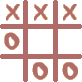Sixth move. Xavier wins the game by placing an X in cell 9, thereby extending the square along the 9th dimension until it becomes a cube. The winning cube number is 16179 or X1101X0X0.

Sound familiar? This is the very room we began our journey from in the Nine-Space Hotel. To anyone who made it all the way to the end of this very long, very strange page, I salute you. I hope you will join me in the exploration of higher space. And if you ever lose your way, I hope Cartan's Triangle will help you find your way home.